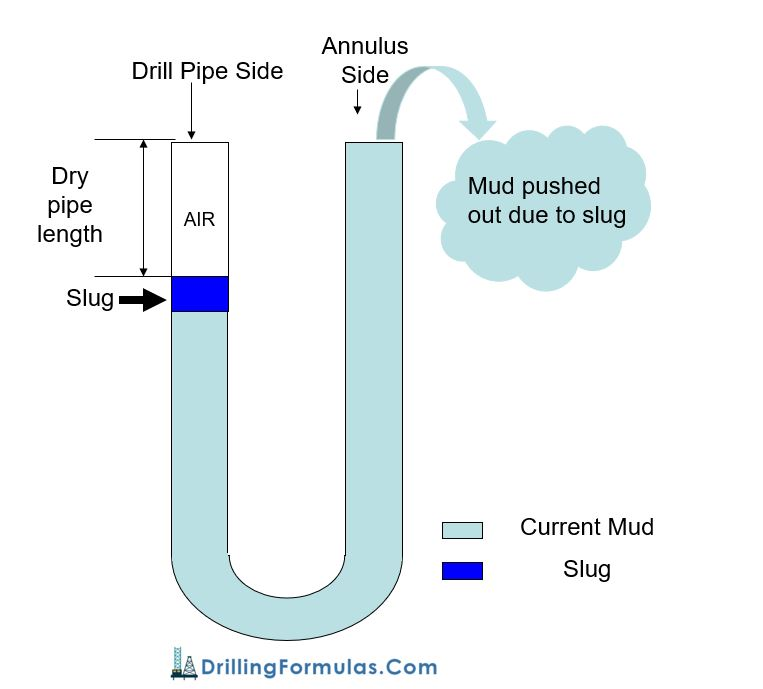# Volume Gain from Slug

Slug mud is typically pumped into the drill string in order to push the mud inside the drill pipe down so the drill pipe will be clean and ready for pulling out of hole. Since slug is heavier, it will push the lighter mud out of the well. Figure 1 demonstrates how slug displaces current mud out of hole.Figure 1 – Diagram Shows Volume Gain from Slug

The volume of mud pushed out of the well, typically called “volume gain from slug” can be determined by the equation below;

Volume Gain from Slug = Slug Volume × (Slug Weight – Current Mud Weight) ÷ Current Mud Weight

Calculation Oilfield Unit

Units used in oilfield are listed below;

• Volume Gain from Slug in bbl
• Slug Volume in bbl
• Slug Weight in ppg
• Current Mud Weight in ppg

Example: Determine volume gain from slug with the following parameters.

Volume slug = 30 bbl
Current mud weight = 12 ppg
Slug weight = 15 ppg

Volume Gain from Slug = 30 × (15- 12) ÷ 12
Volume Gain from Slug = 7.5 bbl

Calculation Metric Unit

Units used in metric are listed below;

• Volume Gain from Slug in
• Slug Volume in
• Slug Weight in kg/m³
• Current Mud Weight in kg/m³

Example: Determine volume gain from slug with the following parameters.

Volume slug = 5 m³
Current mud weight = 1,440 kg/m³
Slug weight = 1,800 kg/m³

Volume Gain from Slug = 5 × (1,800 – 1,440) ÷ 1,440
Volume Gain from Slug = 1.3 m³

The calculation sheet is provided by checking the image below.  This spreadsheet can be modified to suit your work too.Share the joy
Tagged , . Bookmark the permalink.